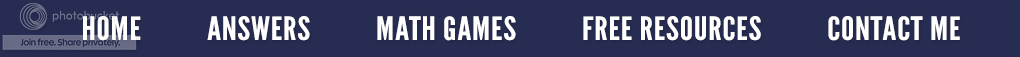### It's A Square Deal

My math-a-magical powers are back.  In my January 23rd post, I demonstrated how to easily divide by the decimal .25.  Today let's look put on our magician's hat and learn how to square any two digit number that ends in 5.

In the number below, look at the digit in the tens place (3) and count up one more.  In this case when we count up, the 3 becomes a 4.  Now multiply the two numbers together, 4 × 3 which equals 12.  Next do 52 which is 5 × 5 = 25.  Therefore, the answer to 352 is 1,225.

Let's try another one.  Remember, it must be a two digit number that ends in 5 for the magic trick to work. This time let's start with 65 and follow the exact same procedure used above.

Did you get the right answer?  Have your students try this using all the two digits numbers that end in 5, beginning with 15 and finishing with 95.  Since all math is based on patterns, ask hem to carefully look for a pattern and describe it.  I think you will be very surprised at what you find!